# Cyclic Redundancy Check | CRC | Example

## Error Detection in Computer Networks-

 Error detection is a technique that is used to check if any error occurred in the data during the transmission.

Some popular error detection methods are-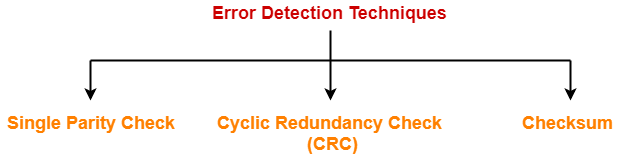1. Single Parity Check
2. Cyclic Redundancy Check (CRC)
3. Checksum

## Cyclic Redundancy Check-

• Cyclic Redundancy Check (CRC) is an error detection method.
• It is based on binary division.

## CRC Generator-

• CRC generator is an algebraic polynomial represented as a bit pattern.
• Bit pattern is obtained from the CRC generator using the following rule-

 The power of each term gives the position of the bit and the coefficient gives the value of the bit.

## Example-

Consider the CRC generator is x7 + x6 + x4 + x3 + x + 1.

The corresponding binary pattern is obtained as-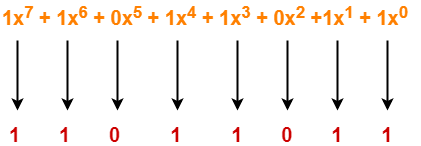Thus, for the given CRC generator, the corresponding binary pattern is 11011011.

## Properties Of CRC Generator-

The algebraic polynomial chosen as a CRC generator should have at least the following properties-

### Rule-01:

• It should not be divisible by x.
• This condition guarantees that all the burst errors of length equal to the length of polynomial are detected.

### Rule-02:

• It should be divisible by x+1.
• This condition guarantees that all the burst errors affecting an odd number of bits are detected.

## Important Notes-

If the CRC generator is chosen according to the above rules, then-

• CRC can detect all single-bit errors
• CRC can detect all double-bit errors provided the divisor contains at least three logic 1’s.
• CRC can detect any odd number of errors provided the divisor is a factor of x+1.
• CRC can detect all burst error of length less than the degree of the polynomial.
• CRC can detect most of the larger burst errors with a high probability.

## Steps Involved-

Error detection using CRC technique involves the following steps-

### Step-01: Calculation Of CRC At Sender Side-

At sender side,

• A string of n 0’s is appended to the data unit to be transmitted.
• Here, n is one less than the number of bits in CRC generator.
• Binary division is performed of the resultant string with the CRC generator.
• After division, the remainder so obtained is called as CRC.
• It may be noted that CRC also consists of n bits.

### Step-02: Appending CRC To Data Unit-

At sender side,

• The CRC is obtained after the binary division.
• The string of n 0’s appended to the data unit earlier is replaced by the CRC remainder.

• The newly formed code word (Original data + CRC) is transmitted to the receiver.

### Step-04: Checking at Receiver Side-

• The transmitted code word is received.
• The received code word is divided with the same CRC generator.
• On division, the remainder so obtained is checked.

The following two cases are possible-

### Case-01: Remainder = 0

If the remainder is zero,

• Receiver assumes that no error occurred in the data during the transmission.

### Case-02: Remainder ≠ 0

If the remainder is non-zero,

• Receiver assumes that some error occurred in the data during the transmission.

## Problem-01:

A bit stream 1101011011 is transmitted using the standard CRC method. The generator polynomial is x4+x+1. What is the actual bit string transmitted?

## Solution-

• The generator polynomial G(x) = x4 + x + 1 is encoded as 10011.
• Clearly, the generator polynomial consists of 5 bits.
• So, a string of 4 zeroes is appended to the bit stream to be transmitted.
• The resulting bit stream is 11010110110000.

Now, the binary division is performed as-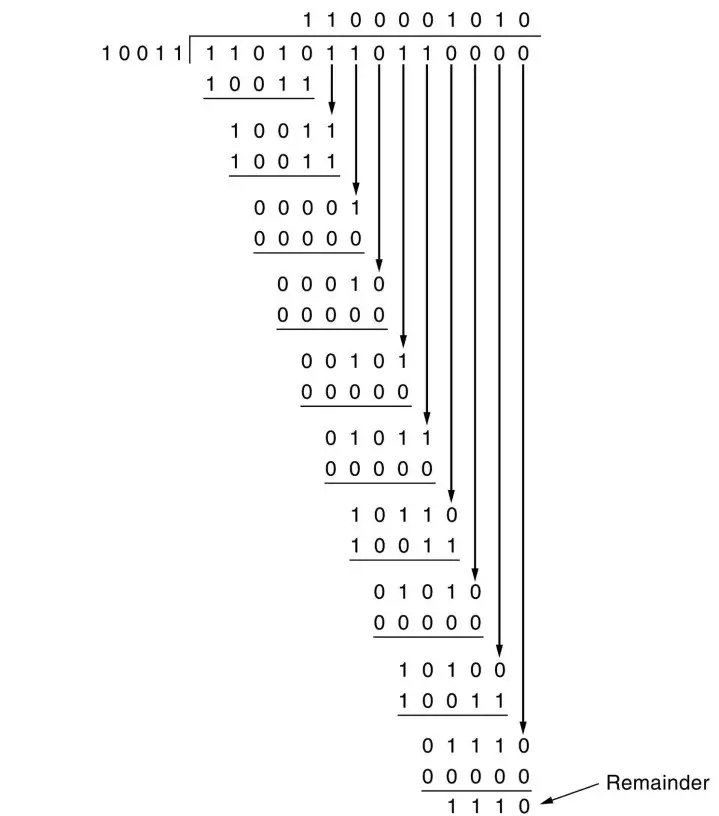From here, CRC = 1110.

Now,

• The code word to be transmitted is obtained by replacing the last 4 zeroes of 11010110110000 with the CRC.
• Thus, the code word transmitted to the receiver = 11010110111110.

## Problem-02:

A bit stream 10011101 is transmitted using the standard CRC method. The generator polynomial is x3+1.

1. What is the actual bit string transmitted?
2. Suppose the third bit from the left is inverted during transmission. How will receiver detect this error?

## Solution-

### Part-01:

• The generator polynomial G(x) = x3 + 1 is encoded as 1001.
• Clearly, the generator polynomial consists of 4 bits.
• So, a string of 3 zeroes is appended to the bit stream to be transmitted.
• The resulting bit stream is 10011101000.

Now, the binary division is performed as-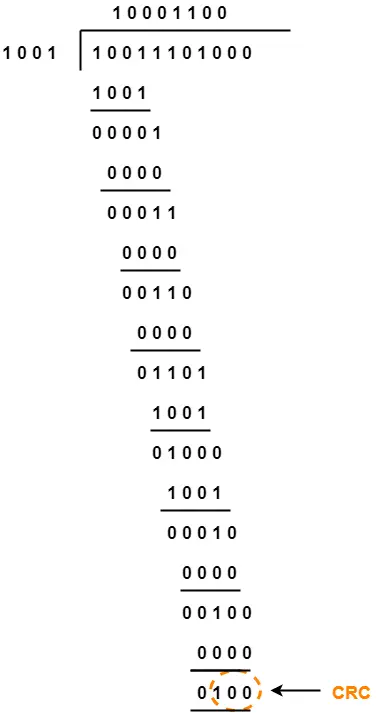From here, CRC = 100.

Now,

• The code word to be transmitted is obtained by replacing the last 3 zeroes of 10011101000 with the CRC.
• Thus, the code word transmitted to the receiver = 10011101100.

## Part-02:

According to the question,

• Third bit from the left gets inverted during transmission.

Now,

• Receiver performs the binary division with the same generator polynomial as-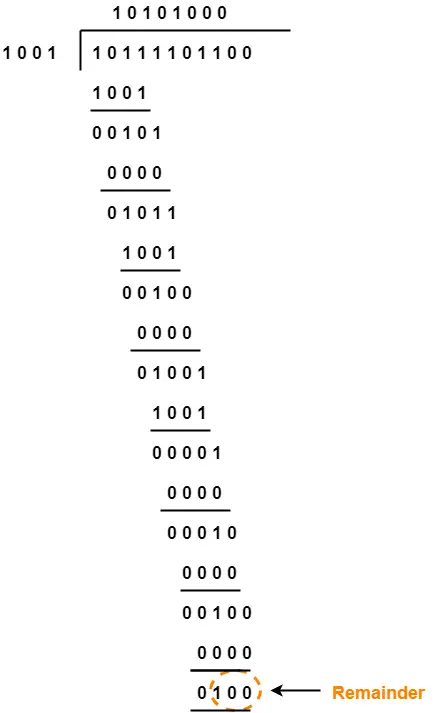From here,

• The remainder obtained on division is a non-zero value.
• This indicates to the receiver that an error occurred in the data during the transmission.
• Therefore, receiver rejects the data and asks the sender for retransmission.

To watch video solution, click here.

To gain better understanding about Cyclic Redundancy Check,

Watch this Video Lecture

Next Article- Checksum

Get more notes and other study material of Computer Networks.

Watch video lectures by visiting our YouTube channel LearnVidFun.

SummaryArticle Name
Cyclic Redundancy Check | CRC | Example
Description
CRC or Cyclic Redundancy Check in computer networks is an error detection method. CRC Generator is used to generate CRC. Example of Cyclic Redundancy Check. CRC or Cyclic Redundancy Check is based on binary division.
Author
Publisher Name
Gate Vidyalay
Publisher Logo